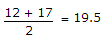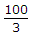# Civil Engineering - UPSC Civil Service Exam Questions

36.

What is the permissible tensile stress is bolts used for column bases ?(Where fy is the yield stress of the steel)

 A. 120 N/mm2 B. 150 N/mm2 C. 0.6 fy D. 0.4 fy

Explanation:

No answer description available for this question. Let us discuss.

37.

King closers are related to

 A. doors and windows B. king post truss C. queen post truss D. bricks masonry

Explanation:

No answer description available for this question. Let us discuss.

38.

The area of a plot is to be determined using Simpson's rule. The following offsets were taken to a boundary from points along a chain line all measurements being in metres 12, 15, 22, 29, 36, 38, 31, 22, 17.There were taken at 100 m intervals.
Consider the following steps in this regard.
1.2. Sum of the odd ordinates = 22 + 36 + 1 = 89
3. Sum of the even ordinates = 15 + 29 + 38 + 22 = 104
4. 12 + 17 = 39
5. Area = 100 (19.5 + 89 + 104)
6. Area =(39 + 2 x 89 + 4 x 104)
7. Area 100 x 19.5 (104 - 89)
The area of the plot can be determined by using the steps listed above :

 A. 1, 2, 3 and 5 B. 1, 3, 4 and 6 C. 1, 2, 4 and 7 D. 2, 3, 4 and 6

Explanation:

No answer description available for this question. Let us discuss.

39.

At a cross-section of a beam, the maximum compressive stress at top is 800 kg/cm2 and its maximum tensile stress at bottom is 200 kg/cm2. The depth of cross-section is 10 cm. The depth of the neutral axis from the top of the section is n. The n is equal to

 A. 2 cm B. 4 cm C. 6 cm D. 8 cm

Explanation:

No answer description available for this question. Let us discuss.

40.

A buttress in a wall is intended to provide

 A. lateral support to roof slab only B. lateral support to wall C. to resist vertical loads only D. lateral support to roof beams only